Anzeige

# rsa-1

15. Jul 2012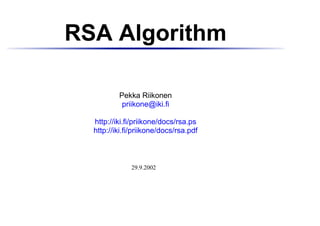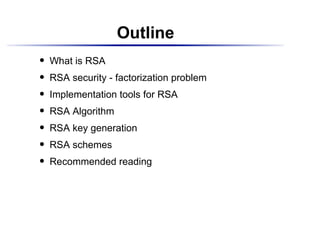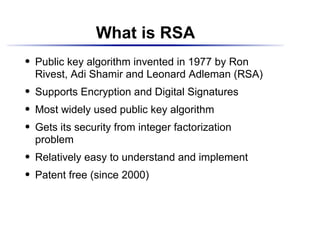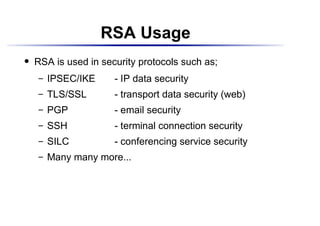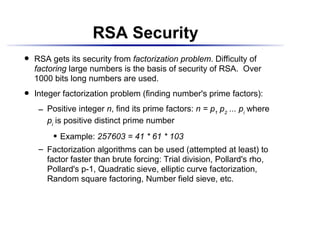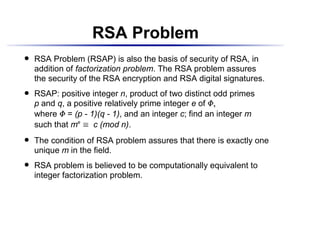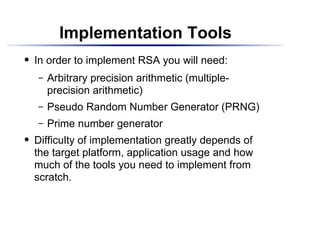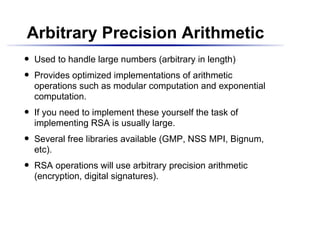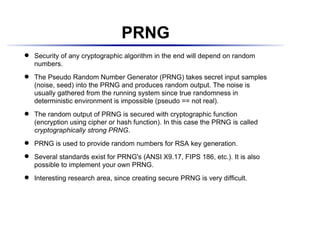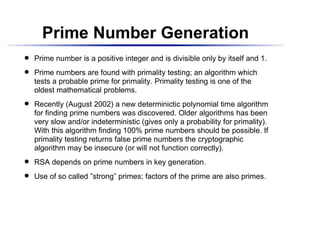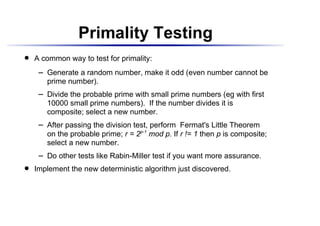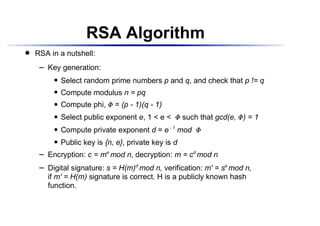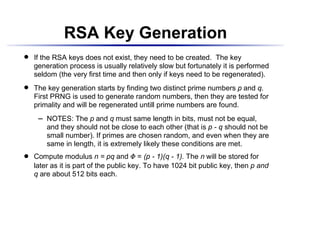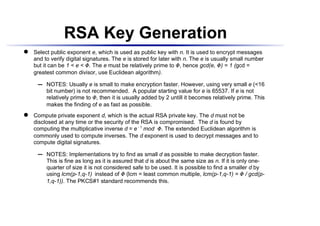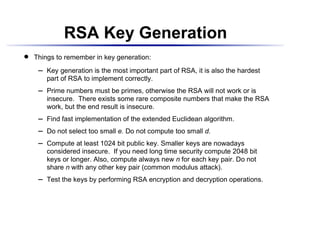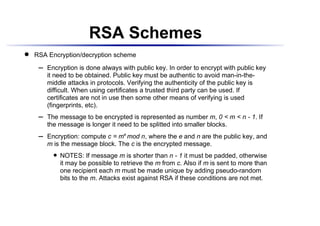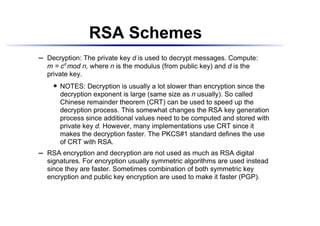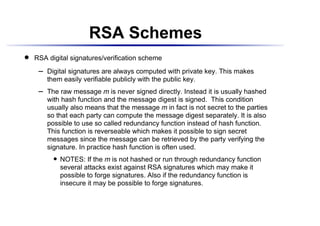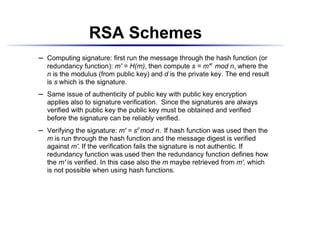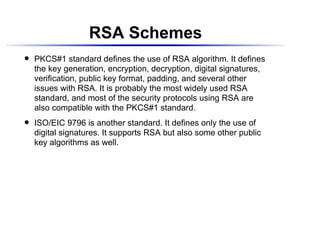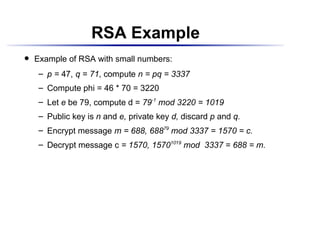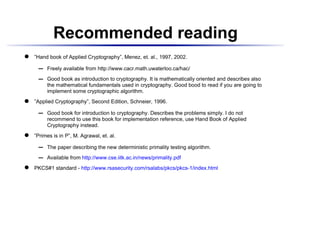1 von 22
Anzeige

### rsa-1

1. RSA Algorithm Pekka Riikonen priikone@iki.fi http://iki.fi/priikone/docs/rsa.ps http://iki.fi/priikone/docs/rsa.pdf 29.9.2002
2. Outline What is RSA   RSA security - factorization problem   Implementation tools for RSA   RSA Algorithm   RSA key generation   RSA schemes   Recommended reading
3. What is RSA Public key algorithm invented in 1977 by Ron   Rivest, Adi Shamir and Leonard Adleman (RSA) Supports Encryption and Digital Signatures   Most widely used public key algorithm   Gets its security from integer factorization   problem Relatively easy to understand and implement   Patent free (since 2000)
4. RSA Usage RSA is used in security protocols such as;   IPSEC/IKE - IP data security   TLS/SSL - transport data security (web)   PGP - email security   SSH - terminal connection security   SILC - conferencing service security   Many many more...
5. RSA Security RSA gets its security from factorization problem. Difficulty of   factoring large numbers is the basis of security of RSA. Over 1000 bits long numbers are used. Integer factorization problem (finding number's prime factors):   Positive integer n, find its prime factors: n = p1 p2 ... pi where   pi is positive distinct prime number Example: 257603 = 41 * 61 * 103   Factorization algorithms can be used (attempted at least) to   factor faster than brute forcing: Trial division, Pollard's rho, Pollard's p-1, Quadratic sieve, elliptic curve factorization, Random square factoring, Number field sieve, etc.
6. RSA Problem RSA Problem (RSAP) is also the basis of security of RSA, in   addition of factorization problem. The RSA problem assures the security of the RSA encryption and RSA digital signatures. RSAP: positive integer n, product of two distinct odd primes   p and q, a positive relatively prime integer e of ¢ ¡ where = (p - 1)(q - 1), and an integer c; find an integer m ¢ such that me c (mod n). £ The condition of RSA problem assures that there is exactly one   unique m in the field. RSA problem is believed to be computationally equivalent to   integer factorization problem.
7. Implementation Tools In order to implement RSA you will need:   Arbitrary precision arithmetic (multiple-   precision arithmetic) Pseudo Random Number Generator (PRNG)   Prime number generator   Difficulty of implementation greatly depends of   the target platform, application usage and how much of the tools you need to implement from scratch.
8. Arbitrary Precision Arithmetic Used to handle large numbers (arbitrary in length)   Provides optimized implementations of arithmetic   operations such as modular computation and exponential computation. If you need to implement these yourself the task of   implementing RSA is usually large. Several free libraries available (GMP, NSS MPI, Bignum,   etc). RSA operations will use arbitrary precision arithmetic   (encryption, digital signatures).
9. PRNG Security of any cryptographic algorithm in the end will depend on random   numbers. The Pseudo Random Number Generator (PRNG) takes secret input samples   (noise, seed) into the PRNG and produces random output. The noise is usually gathered from the running system since true randomness in deterministic environment is impossible (pseudo == not real). The random output of PRNG is secured with cryptographic function   (encryption using cipher or hash function). In this case the PRNG is called cryptographically strong PRNG. PRNG is used to provide random numbers for RSA key generation.   Several standards exist for PRNG's (ANSI X9.17, FIPS 186, etc.). It is also   possible to implement your own PRNG. Interesting research area, since creating secure PRNG is very difficult.
10. Prime Number Generation Prime number is a positive integer and is divisible only by itself and 1.   Prime numbers are found with primality testing; an algorithm which   tests a probable prime for primality. Primality testing is one of the oldest mathematical problems. Recently (August 2002) a new determinictic polynomial time algorithm   for finding prime numbers was discovered. Older algorithms has been very slow and/or indeterministic (gives only a probability for primality). With this algorithm finding 100% prime numbers should be possible. If primality testing returns false prime numbers the cryptographic algorithm may be insecure (or will not function correctly). RSA depends on prime numbers in key generation.   Use of so called ”strong” primes; factors of the prime are also primes.
11. Primality Testing A common way to test for primality:   Generate a random number, make it odd (even number cannot be   prime number). Divide the probable prime with small prime numbers (eg with first   10000 small prime numbers). If the number divides it is composite; select a new number. After passing the division test, perform Fermat's Little Theorem   on the probable prime; r = 2p-1 mod p. If r != 1 then p is composite; select a new number. Do other tests like Rabin-Miller test if you want more assurance.   Implement the new deterministic algorithm just discovered.
12. RSA Algorithm RSA in a nutshell:   Key generation:   Select random prime numbers p and q, and check that p != q ¡ Compute modulus n = pq ¡ Compute phi, = (p - 1)(q - 1) ¢ ¡ Select public exponent e, 1 < e < such that gcd(e, ) = 1 ¢ ¢ ¡ Compute private exponent d = e - 1 mod ¢ ¡ Public key is {n, e}, private key is d ¡ Encryption: c = me mod n, decryption: m = cd mod n   Digital signature: s = H(m)d mod n, verification: m' = se mod n,   if m' = H(m) signature is correct. H is a publicly known hash function.
13. RSA Key Generation If the RSA keys does not exist, they need to be created. The key   generation process is usually relatively slow but fortunately it is performed seldom (the very first time and then only if keys need to be regenerated). The key generation starts by finding two distinct prime numbers p and q.   First PRNG is used to generate random numbers, then they are tested for primality and will be regenerated untill prime numbers are found. NOTES: The p and q must same length in bits, must not be equal,   and they should not be close to each other (that is p - q should not be small number). If primes are chosen random, and even when they are same in length, it is extremely likely these conditions are met. Compute modulus n = pq and = (p - 1)(q - 1). The n will be stored for ¢   later as it is part of the public key. To have 1024 bit public key, then p and q are about 512 bits each.
14. RSA Key Generation   Select public exponent e, which is used as public key with n. It is used to encrypt messages and to verify digital signatures. The e is stored for later with n. The e is usually small number but it can be 1 < e < . The e must be relatively prime to , hence gcd(e, ) = 1 (gcd =       greatest common divisor, use Euclidean algorithm). NOTES: Usually e is small to make encryption faster. However, using very small e (<16 ¡ bit number) is not recommended. A popular starting value for e is 65537. If e is not relatively prime to , then it is usually added by 2 untill it becomes relatively prime. This   makes the finding of e as fast as possible.   Compute private exponent d, which is the actual RSA private key. The d must not be disclosed at any time or the security of the RSA is compromised. The d is found by computing the multiplicative inverse d = e - 1 mod . The extended Euclidean algorithm is   commonly used to compute inverses. The d exponent is used to decrypt messages and to compute digital signatures. NOTES: Implementations try to find as small d as possible to make decryption faster. ¡ This is fine as long as it is assured that d is about the same size as n. If it is only one- quarter of size it is not considered safe to be used. It is possible to find a smaller d by using lcm(p-1,q-1) instead of (lcm = least common multiple, lcm(p-1,q-1) = / gcd(p-     1,q-1)). The PKCS#1 standard recommends this.
15. RSA Key Generation Things to remember in key generation:   Key generation is the most important part of RSA, it is also the hardest ¡ part of RSA to implement correctly. Prime numbers must be primes, otherwise the RSA will not work or is ¡ insecure. There exists some rare composite numbers that make the RSA work, but the end result is insecure. Find fast implementation of the extended Euclidean algorithm. ¡ Do not select too small e. Do not compute too small d. ¡ Compute at least 1024 bit public key. Smaller keys are nowadays ¡ considered insecure. If you need long time security compute 2048 bit keys or longer. Also, compute always new n for each key pair. Do not share n with any other key pair (common modulus attack). Test the keys by performing RSA encryption and decryption operations. ¡
16. RSA Schemes RSA Encryption/decryption scheme   Encryption is done always with public key. In order to encrypt with public key ¡ it need to be obtained. Public key must be authentic to avoid man-in-the- middle attacks in protocols. Verifying the authenticity of the public key is difficult. When using certificates a trusted third party can be used. If certificates are not in use then some other means of verifying is used (fingerprints, etc). The message to be encrypted is represented as number m, 0 < m < n - 1. If ¡ the message is longer it need to be splitted into smaller blocks. Encryption: compute c = me mod n, where the e and n are the public key, and ¡ m is the message block. The c is the encrypted message. NOTES: If message m is shorter than n - 1 it must be padded, otherwise   it may be possible to retrieve the m from c. Also if m is sent to more than one recipient each m must be made unique by adding pseudo-random bits to the m. Attacks exist against RSA if these conditions are not met.
17. RSA Schemes Decryption: The private key d is used to decrypt messages. Compute: ¡ m = cd mod n, where n is the modulus (from public key) and d is the private key. NOTES: Decryption is usually a lot slower than encryption since the   decryption exponent is large (same size as n usually). So called Chinese remainder theorem (CRT) can be used to speed up the decryption process. This somewhat changes the RSA key generation process since additional values need to be computed and stored with private key d. However, many implementations use CRT since it makes the decryption faster. The PKCS#1 standard defines the use of CRT with RSA. RSA encryption and decryption are not used as much as RSA digital ¡ signatures. For encryption usually symmetric algorithms are used instead since they are faster. Sometimes combination of both symmetric key encryption and public key encryption are used to make it faster (PGP).
18. RSA Schemes RSA digital signatures/verification scheme   Digital signatures are always computed with private key. This makes ¡ them easily verifiable publicly with the public key. The raw message m is never signed directly. Instead it is usually hashed ¡ with hash function and the message digest is signed. This condition usually also means that the message m in fact is not secret to the parties so that each party can compute the message digest separately. It is also possible to use so called redundancy function instead of hash function. This function is reverseable which makes it possible to sign secret messages since the message can be retrieved by the party verifying the signature. In practice hash function is often used. NOTES: If the m is not hashed or run through redundancy function   several attacks exist against RSA signatures which may make it possible to forge signatures. Also if the redundancy function is insecure it may be possible to forge signatures.
19. RSA Schemes Computing signature: first run the message through the hash function (or ¡ redundancy function): m' = H(m), then compute s = m'd mod n, where the n is the modulus (from public key) and d is the private key. The end result is s which is the signature. Same issue of authenticity of public key with public key encryption ¡ applies also to signature verification. Since the signatures are always verified with public key the public key must be obtained and verified before the signature can be reliably verified. Verifying the signature: m' = sd mod n. If hash function was used then the ¡ m is run through the hash function and the message digest is verified against m'. If the verification fails the signature is not authentic. If redundancy function was used then the redundancy function defines how the m' is verified. In this case also the m maybe retrieved from m', which is not possible when using hash functions.
20. RSA Schemes PKCS#1 standard defines the use of RSA algorithm. It defines   the key generation, encryption, decryption, digital signatures, verification, public key format, padding, and several other issues with RSA. It is probably the most widely used RSA standard, and most of the security protocols using RSA are also compatible with the PKCS#1 standard. ISO/EIC 9796 is another standard. It defines only the use of   digital signatures. It supports RSA but also some other public key algorithms as well.
21. RSA Example Example of RSA with small numbers:   p = 47, q = 71, compute n = pq = 3337 ¡ Compute phi = 46 * 70 = 3220 ¡ Let e be 79, compute d = 79-1 mod 3220 = 1019 ¡ Public key is n and e, private key d, discard p and q. ¡ Encrypt message m = 688, 68879 mod 3337 = 1570 = c. ¡ Decrypt message c = 1570, 15701019 mod 3337 = 688 = m. ¡
22. Recommended reading   ”Hand book of Applied Cryptography”, Menez, et. al., 1997, 2002. Freely available from http://www.cacr.math.uwaterloo.ca/hac/ ¡ Good book as introduction to cryptography. It is mathematically oriented and describes also ¡ the mathematical fundamentals used in cryptography. Good bood to read if you are going to implement some cryptographic algorithm.   ”Applied Cryptography”, Second Edition, Schneier, 1996. Good book for introduction to cryptography. Describes the problems simply. I do not ¡ recommend to use this book for implementation reference, use Hand Book of Applied Cryptography instead.   ”Primes is in P”, M. Agrawal, et. al. The paper describing the new deterministic primality testing algorithm. ¡ Available from http://www.cse.iitk.ac.in/news/primality.pdf ¡   PKCS#1 standard - http://www.rsasecurity.com/rsalabs/pkcs/pkcs-1/index.html
Anzeige